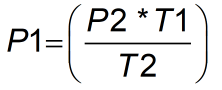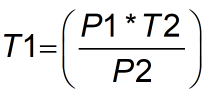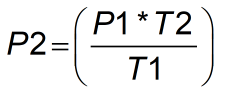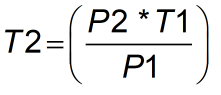Request a Tool

Gay Lussacs Law Calculator

Gay-Lussac's law is an ideal gas law where at constant volume , the pressure of an ideal gas is directly proportional to its absolute temperature

Output

Initial Pressure (P1)
0

Output

Intial Temperture (T1)
0

Output

Final Pressure (P2)
0

Output

Final Temperature (T2)
0

Formula• P1 = initial pressure
• T1 = initial temperature
• P2 = final pressure
• T2 = final temperature

Defination / Uses

Gay-Lussac's law is an ideal gas law where at constant volume , the pressure of an ideal gas is directly proportional to its absolute temperature. In other words, the law states the principle that, for relatively low pressures, the density of an ideal gas at constant pressure varies inversely with the absolute temperature of the gas.Unit of Pressure is denoted as kPa and temperature is kelvin K.

Formula• P1 = initial pressure
• T1 = initial temperature
• P2 = final pressure
• T2 = final temperature

Defination / Uses

Gay-Lussac's law is an ideal gas law where at constant volume , the pressure of an ideal gas is directly proportional to its absolute temperature. In other words, the law states the principle that, for relatively low pressures, the density of an ideal gas at constant pressure varies inversely with the absolute temperature of the gas.Unit of Pressure is denoted as kPa and temperature is kelvin K.

Formula• P1 = initial pressure
• T1 = initial temperature
• P2 = final pressure
• T2 = final temperature

Defination / Uses

Gay-Lussac's law is an ideal gas law where at constant volume , the pressure of an ideal gas is directly proportional to its absolute temperature. In other words, the law states the principle that, for relatively low pressures, the density of an ideal gas at constant pressure varies inversely with the absolute temperature of the gas.Unit of Pressure is denoted as kPa and temperature is kelvin K.

Formula• P1 = initial pressure
• T1 = initial temperature
• P2 = final pressure
• T2 = final temperature

Defination / Uses

Gay-Lussac's law is an ideal gas law where at constant volume , the pressure of an ideal gas is directly proportional to its absolute temperature. In other words, the law states the principle that, for relatively low pressures, the density of an ideal gas at constant pressure varies inversely with the absolute temperature of the gas.Unit of Pressure is denoted as kPa and temperature is kelvin K.

During an isochoric transition, this Gay-Lussac's law calculator will give you information on the basic gas parameters. In the late 18th and early 19th centuries, French chemist Joseph Louis Gay-Lussac (1778–1850) and other scientists made significant discoveries on thermal expansion of gases and the link between temperature, volume, and pressure, which became known as Gay-Lussac's law. The pressure of a gas is directly proportional to its temperature for a given amount of gas and at constant volume, according to Gay-Lussac's law. If a gas's temperature rises, its pressure rises as well, assuming the gas's mass and volume remain unchanged. Use the upper given formula for manual calculations. No sign-up, registration OR captcha is required to use this tool.